Courses

# Z-Transform - 2

## 20 Questions MCQ Test Topicwise Question Bank for Electrical Engineering | Z-Transform - 2

Description
This mock test of Z-Transform - 2 for Electrical Engineering (EE) helps you for every Electrical Engineering (EE) entrance exam. This contains 20 Multiple Choice Questions for Electrical Engineering (EE) Z-Transform - 2 (mcq) to study with solutions a complete question bank. The solved questions answers in this Z-Transform - 2 quiz give you a good mix of easy questions and tough questions. Electrical Engineering (EE) students definitely take this Z-Transform - 2 exercise for a better result in the exam. You can find other Z-Transform - 2 extra questions, long questions & short questions for Electrical Engineering (EE) on EduRev as well by searching above.
QUESTION: 1

Solution:
QUESTION: 2

Solution:
QUESTION: 3

### If, the ROC of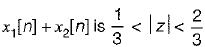then the ROC of x1[n]-x2[n] includes

Solution:
QUESTION: 4

The z-transform of discrete time signal x[n] = {1, 0, -1,0, 1,-1} is

Solution:
QUESTION: 5

A all pass causal LTI system is described by the difference equation:
y[n] - ay[n - 1] = bx[n] + x [n - 1]
The value of ’b’ will be

Solution:
QUESTION: 6

Consider a discrete time signal x(n) having z-transform given by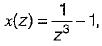if x(n) is right sided then z-transform x(z) belongs to

Solution:
QUESTION: 7

Consider a system with system function: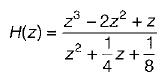Solution:
QUESTION: 8

Let x[n] =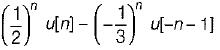The ROC for the z-transform of above sequence is

Solution:
QUESTION: 9

The sequence x[n] corresponding tox (z) defined as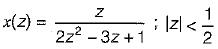will have the value at n = - 2

Solution:
QUESTION: 10

The direct.form-II realisation of []R filter is shown below.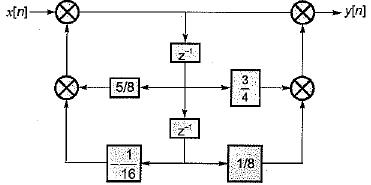The transfer function is

Solution:
QUESTION: 11

A sequence of x(n) with the z-transform x(z) = z4 + z2 - 2z + 2 - 3z-4 is applied as an input to a L.T.I system with the impulse response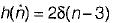where,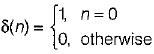the output at n = 2 is

Solution:
QUESTION: 12

Find x[n] for the case, when x(z) =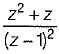Solution:
QUESTION: 13

Match List-I {x(n)} with List-II {x(z)} and select the correct answer using the codes given below the lists:
List-I
A. ∝n u(n)
B. ∝n u(-n -1)
C. ∝n u(-n-1)
D. ∝u(n)
List-II
1.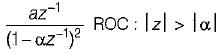2.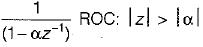3.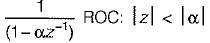4.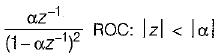Codes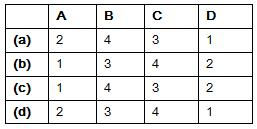Solution:
QUESTION: 14

Choose the correct option for given causal systems, stable or not
i.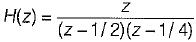ii.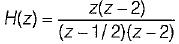iii.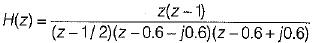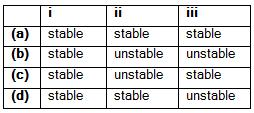Solution:
QUESTION: 15

Find the inverse z-transform of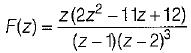Solution:
QUESTION: 16

Consider discrete time signal x(n) is given by
x(n) = 4n u(n)
Also,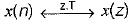Consider another signal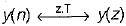Also, y(z) = x2(z)
then, y(n) is

Solution:
QUESTION: 17

Consider a z-transform of a signal x(n)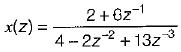value of x(1) is equal to

Solution:
QUESTION: 18

Consider a discrete time signal x(n), who use z-transform x(z) is given by,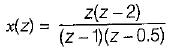value of x(n) for n = 0 is

Solution:
QUESTION: 19

If z-transform is given by x(z) = cos(z-3), |z| > 0 the value of x is

Solution:
QUESTION: 20

The impulse response of the system in below figure.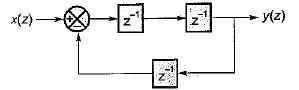Solution: